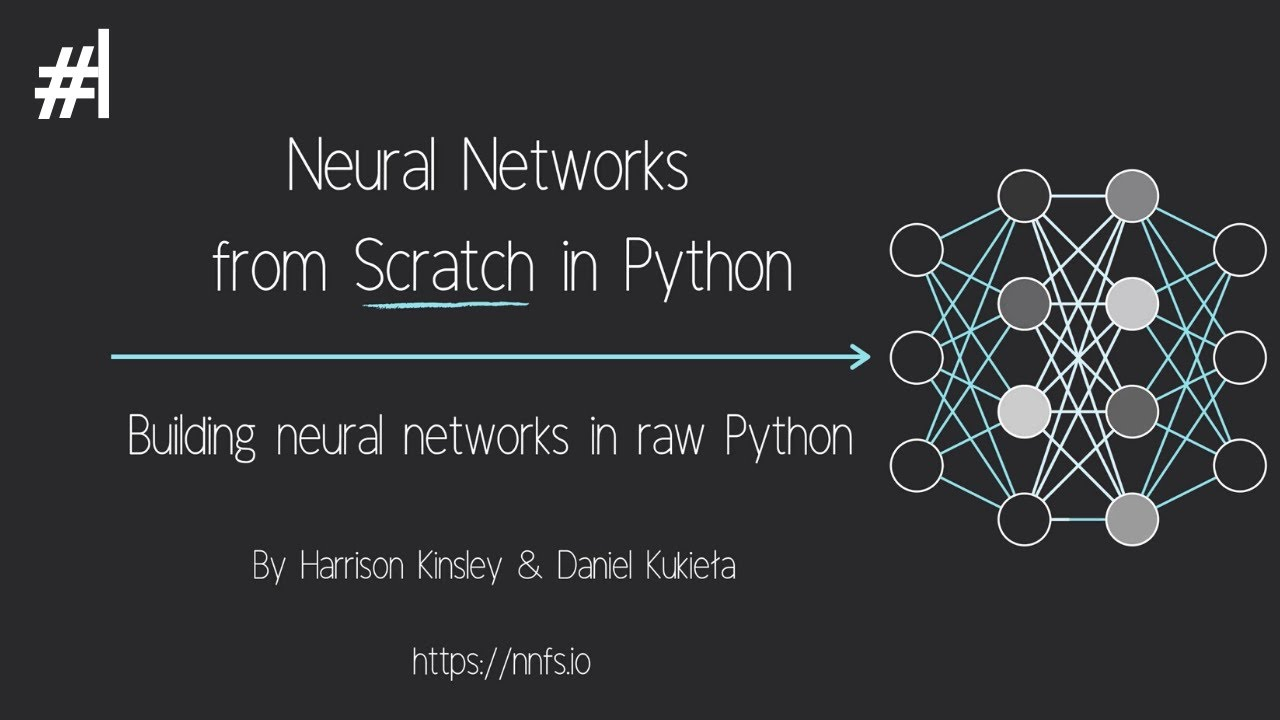# Neural Networks from ScratchBuilding neural networks from scratch in Python introduction. Neural Networks from Scratch book: https://nnfs.io Playlist for this series.

Building neural networks from scratch in Python introduction.

Neural Networks from Scratch book: https://nnfs.io

Python 3 basics: https://pythonprogramming.net/introduction-learn-python-3-tutorials/ Intermediate Python (w/ OOP):https://pythonprogramming.net/introduction-intermediate-python-tutorial/

## Neural network: what is a neural network?

Neural networks, as their name implies, are computer algorithms modeled after networks of neurons in the human brain. Learn more about neural networks from Algorithmia.

## A Comparative Analysis of Recurrent Neural Networks

Recurrent neural networks, also known as RNNs, are a class of neural networks that allow previous outputs to be used as inputs while having hidden states.

## Recurrent Neural Networks for Multilabel Text Classification Tasks

The purpose of this project is to build and evaluate Recurrent Neural Networks(RNNs) for sentence-level classification tasks. Let's understand about recurrent neural networks for multilabel text classification tasks.

## Neural Network on Beer Dataset

Artificial neural networks (ANNs), usually simply called neural networks (NNs), are computing systems vaguely inspired by the biological neural networks that constitute animal brains.

## Convolutional Neural Network: How is it different from the other networks?

Convolutional Neural Network: How is it different from the other networks? What’s so unique about CNNs and what does convolution really do? This is a math-free introduction to the wonders of CNNs.